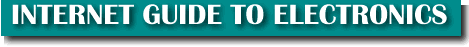BASIC ELECTRONIC CIRCUITS EXPLAINED

In this section we will discuss what a circuit is. I won't belabor the principles of the atom -- let a physics text handle that (boring) task. Instead let's talk about the facts you will need to know to get started in electronics.

Circuit
A circuit is a path for electrons to flow through. The path is from a power sources negative terminal, through the various components and on to the positive terminal.

Think of it as a circle. The paths may split off here and there but they always for a line from the negative to positive.

NOTE: Negatively charged electrons in a conductor are attracted to the positive side of the power source.

Conductor
A conductor is a material (usually a metal such as copper) that allows electrical current to pass easily through. The current is made up of electrons. This is opposed to an insulator which prevents the flow of electricity through it.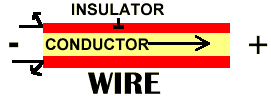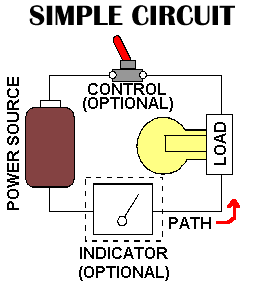Simple Circuit If we break a circuit down to it's elementary blocks we get: 1) A Power Source -- eg: battery 2) A Path -- eg: a wire 3) A Load -- eg: a lamp 4) A Control -- eg: switch (Optional) 5) An indicator -- eg: Meter (Optional)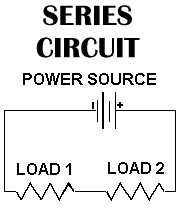Series Circuit A series circuit is one with all the loads in a row. Like links in a chain. There is only ONE path for the electricity to flow. If this circuit was a string of light bulbs, and one blew out, the remaining bulbs would turn off. There is specific properties to this circuit that will be described in another section. NOTE: The squiggly lines in the diagram are the symbol for Resistors. The parallel lines are the symbol for a battery.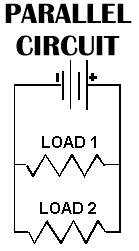Parallel Circuit A parallel circuit is one that has two or more paths for the electricity to flow. In other words, the loads are parallel to each other. If the loads in this circuit were light bulbs and one blew out there is still current flowing to the others as they are still in a direct path from the negative to positive terminals of the battery. There are also specific properties a parallel this circuit that will be described in another section.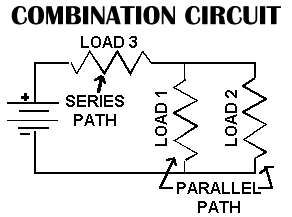Combination Circuit A combination circuit is one that has a "combination" of series and parallel paths for the electricity to flow. Its properties are a synthesis of the two. In this example, the parallel section of the circuit is like a sub-circuit and actually is part of an over-all series circuit.

TOP